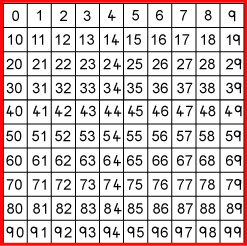#### You may also like### Pebbles

Place four pebbles on the sand in the form of a square. Keep adding as few pebbles as necessary to double the area. How many extra pebbles are added each time?### It Figures

Suppose we allow ourselves to use three numbers less than 10 and multiply them together. How many different products can you find? How do you know you've got them all?### Bracelets

Investigate the different shaped bracelets you could make from 18 different spherical beads. How do they compare if you use 24 beads?

# Give Me Four Clues

##### Age 7 to 11Challenge LevelI am thinking of a number between 1 and 100. What's my number?

Look at the eight clues below.
Can you sort out the four clues that enable you to deduce my number?
Which four clues are not necessary, even though they are true?

Clues:

1. The number is greater than $9$.
2. The number is not a multiple of $10$.
3. The number is a multiple of $7$.
4. The number is odd.
5. The number is not a multiple of $11$.
6. The number is less than $200$.
7. Its ones digit is larger than its tens digit.
8. Its tens digit is odd.

Challenge:
Choose your own secret number between 1 and 100.
Write exactly four clues so that your friend needs each of them to guess your number.
Try it out on your friend.

Remember, avoid clues that double-up and therefore are not needed such as both 'it's even' and 'it's in the 4 times table'. Your four clues must lead to just one number between 1 and 100.